徐小夕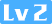241 0 0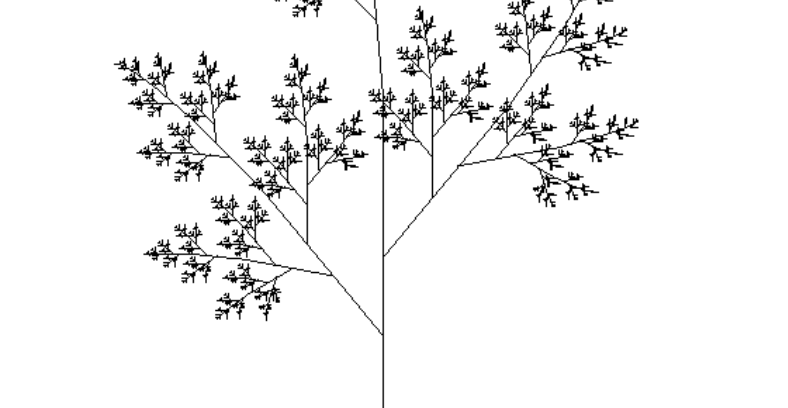### 递归和尾递归

function factorial(n) {
if (n === 1) return 1;
return n * factorial(n - 1);
}

factorial(5) // 120

function factorial(n, total = 1) {
if (n === 1) return total;
return factorial(n - 1, n * total);
}

factorial(5) // 120

### 递归的常用应用案例

#### 1. 数组求和

function sumArray(arr, total) {
if(arr.length === 1) {
return total
}
return sum(arr, total + arr.pop())
}

let arr = [1,2,3,4];
sumArray(arr, arr) // 10

#### 2. 斐波那且数列

// 斐波那契数列
function factorial1 (n) {
if(n <= 2){
return 1
}
return factorial1(n-1) + factorial1(n-2)
}

// 尾递归优化后
function factorial2 (n, start = 1, total = 1) {
if(n <= 2){
return total
}
return factorial2 (n -1, total, total + start)
}

#### 5. 深拷贝

 function clone(target) {
if (typeof target === 'object') {
let cloneTarget = Array.isArray(target) ? [] : {};
for (const key in target) {
cloneTarget[key] = clone(target[key]);
}
return cloneTarget;
} else {
return target;
}
};

#### 6. 爬梯问题

n =1; result = 1  --> 1
n =2; result = 2  --> 11 2
n =3; result = 3  --> 111 12 21
...

function steps(n) {
if(n <= 1) {
return 1
}
return steps(n-1) + steps(n-2)
}

#### 7. 对象数据格式化

let obj = {
a: '1',
b: {
c: '2',
D: {
E: '3'
}
}
}

let obj = {
a: '1',
b: {
c: '2',
d: {
e: '3'
}
}
}

// 代码实现
function keysLower(obj) {
let reg = new RegExp("([A-Z]+)", "g");
for (let key in obj) {
if (obj.hasOwnProperty(key)) {
let temp = obj[key];
if (reg.test(key.toString())) {
// 将修改后的属性名重新赋值给temp，并在对象obj内添加一个转换后的属性
temp = obj[key.replace(reg, function (result) {
return result.toLowerCase()
})] = obj[key];
// 将之前大写的键属性删除
delete obj[key];
}
// 如果属性是对象或者数组，重新执行函数
if (typeof temp === 'object' || Object.prototype.toString.call(temp) === '[object Array]') {
keysLower(temp);
}
}
}
return obj;
};

#### 8. 遍历目录/删除目录

function deleteFolder(path) {
var files = [];
if(fs.existsSync(path)) { // 如果目录存在
files = fs.readdirSync(path);
files.forEach(function(file,index){
var curPath = path + "/" + file;
if(fs.statSync(curPath).isDirectory()) { // 如果是目录，则递归
deleteFolder(curPath);
} else { // 删除文件
fs.unlinkSync(curPath);
}
});
fs.rmdirSync(path);
}
}

#### 9. 绘制分形图形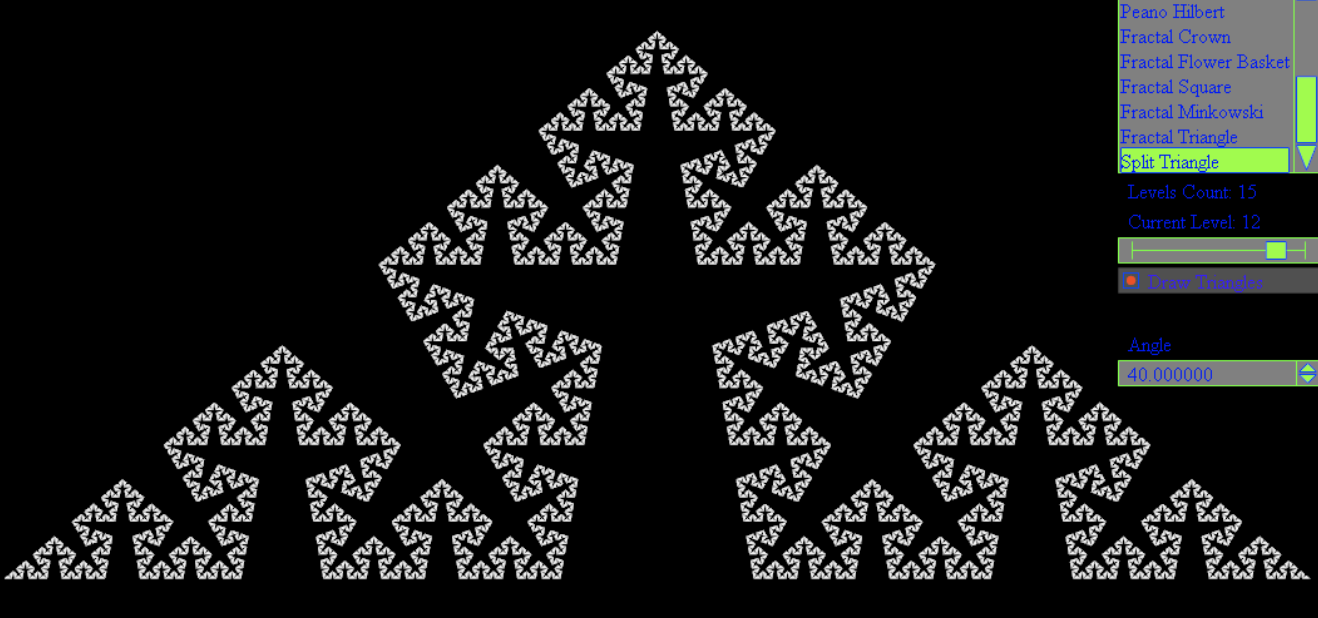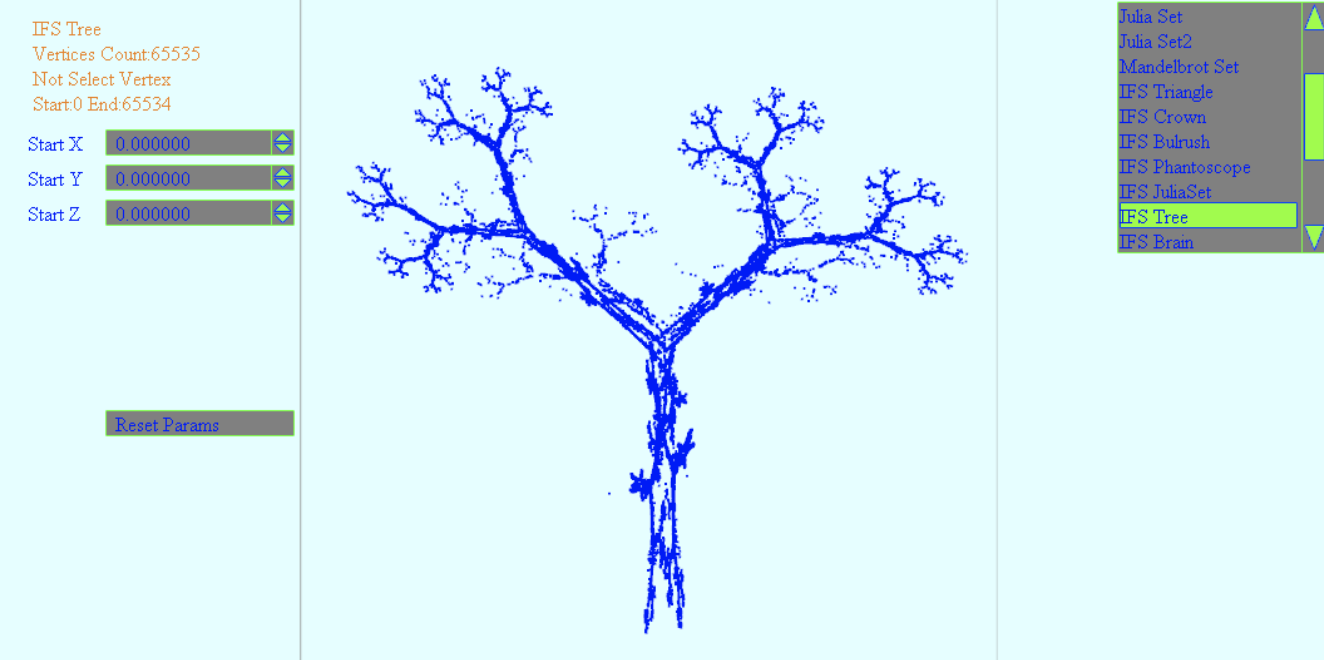我们可以借助一些工具和递归的思想，实现如上的分形图案。

#### 10. 扁平化数组Flat

let a = [1,2,3, [1,2,3, [1,2,3]]]
// 变成
let a = [1,2,3,1,2,3,1,2,3]
// 具体实现
function flat(arr = [], result = []) {
arr.forEach(v => {
if(Array.isArray(v)) {
result = result.concat(flat(v, []))
}else {
result.push(v)
}
})
return result
}

flat(a)

### 用递归画一棵自定义风格的结构树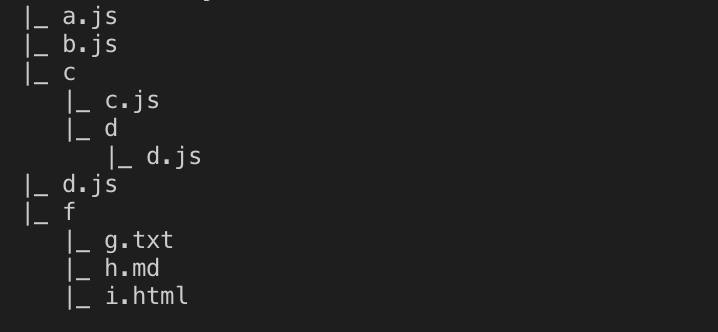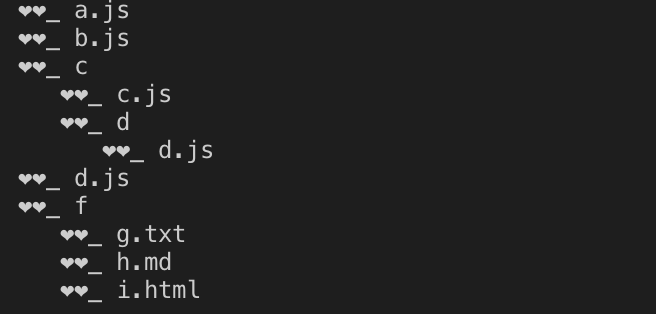该图形是根据目录结构生成的目录树图，在很多应用场景中被广泛使用，接下来我们就来看看他的实现过程吧：

const fs = require('fs')
const path = require('path')
// 遍历目录/生成目录树
function treeFolder(path, flag = '|_') {
var files = [];

if(fs.existsSync(path)) {
files = fs.readdirSync(path);
files.forEach(function(file,index){
var curPath = path + "/" + file;
if(fs.statSync(curPath).isDirectory()) { // recurse
// obj[file] = treeFolder(curPath, {});
console.log(flag, file)
treeFolder(curPath, '   ' + flag)
} else {
// obj['--'] = file
console.log(flag, file)
}
})
// return obj
}
}

treeFolder(path.resolve(__dirname, './test'))

test为我们建的测试目录，如下：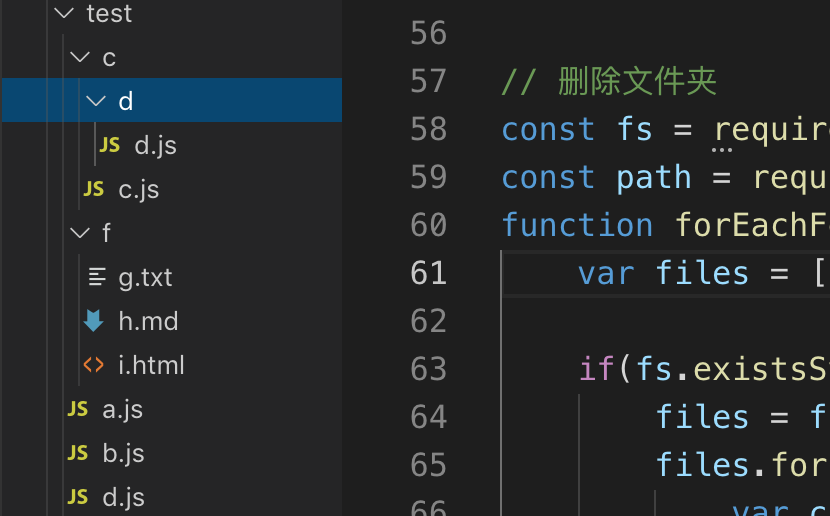我们通过短短10几行代码就实现了一个生成结构树的小应用，是不是感觉递归有点意思呢？在这个函数中，第一个参数是目录的绝对路径，第二个是标示符，标示符决定我们生成的树枝的样式，我们可以自定义不同的样式。